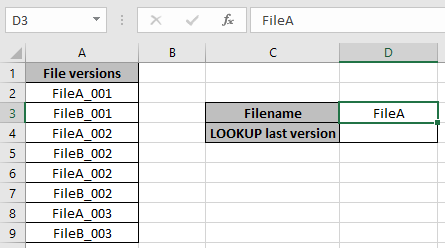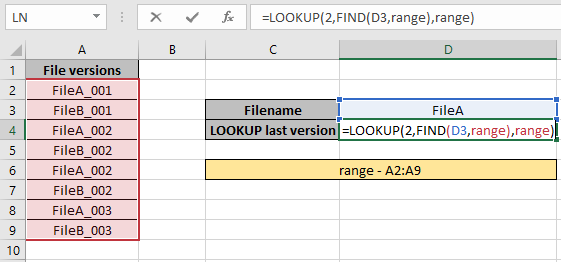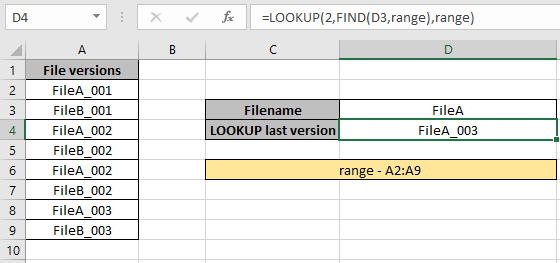# How to LOOKUP file with Last ersion

In this article, we will learn how to get the newest file version using Excel LOOKUP function.

LOOKUP function finds the value in the range and returns the value from the range or its corresponding value. But here we will be using one of the properties of the LOOKUP function i.e. if LOOKUP function can't find an exact match of lookup value, it will match with the last biggest value in the range. (the biggest value, in the range, but smaller than the lookup value).

Generic Formula:

= LOOKUP ( 2 , FIND ( filename , range) , range )

Let’s understand this by using the above stated formula in an example

Here we have file versions in the first column and we need to find the last version for the FileA.Use the formula in the D4 cell:

= LOOKUP ( 2 , FIND(D3 , range) , range)

Explanation:

• If the filename match is found, the FIND function returns 1  for the matched values.
• If the match is not found, then the FIND function returns the #VALUE error. And returns the array of 1's and #VALUE errors.
• Now the lookup function lookup value ( 2 ) in the list of array of 1's and #VALUE! Errors.
• So when the function doesn't find the value 2. So it returns the last value that was the biggest value but less than 2.Here the range is named range for the A2:A9 array.
Press Enter to get the results.As you can see we got the last file version using the LOOKUP & FIND function.

Notes:

1. The formula returns #NA error if no match is found in the array.
2. The formula checks the lookup value first and then looks up for the just previous value, if the lookup value is not found.
3. All excel formula always returns the first value found in the table array.
4. In the above explained formula, look up the last file version in the filename array.

Hope you understood how to use the LOOKUP function in Excel. Explore more articles on lookup value here. Please feel free to state your queries below in the comment box. We will certainly help you.

Related Articles

Use INDEX and MATCH to Lookup Value

How to use the INDEX function in Excel

How to use the MATCH function in Excel

How to use LOOKUP function in Excel

How to use the VLOOKUP function in Excel

How to use the HLOOKUP function in Excel

Popular Articles

Edit a dropdown list

If with conditional formatting

If with wildcards

Vlookup by date

Terms and Conditions of use

The applications/code on this site are distributed as is and without warranties or liability. In no event shall the owner of the copyrights, or the authors of the applications/code be liable for any loss of profit, any problems or any damage resulting from the use or evaluation of the applications/code.